## How to control the current in a LED

As we know, LED is a light-emitting diode which is restricted to its breakover current. If the current value through LED is higher than its limitation, the LED will be damaged. Based on this, LED must connect to its own current limiting series resistor which we can also use for controlling the brightness of LED. In this tutorial, we�ll build a buck converter for LED considering its low required voltage and use a PI controller to control the current of LEDs as below. The idea of PI controller is to measure the current value of the four series LEDs and then compare it with the reference current (If=350mA) for adding a decision of the switch in the buck converter. Since the reference current is 350mA, the voltage of the current limiting series resistor shall be 350mA*2.2 ohm. That�s the grounds that we use a voltage sensor in this schematic.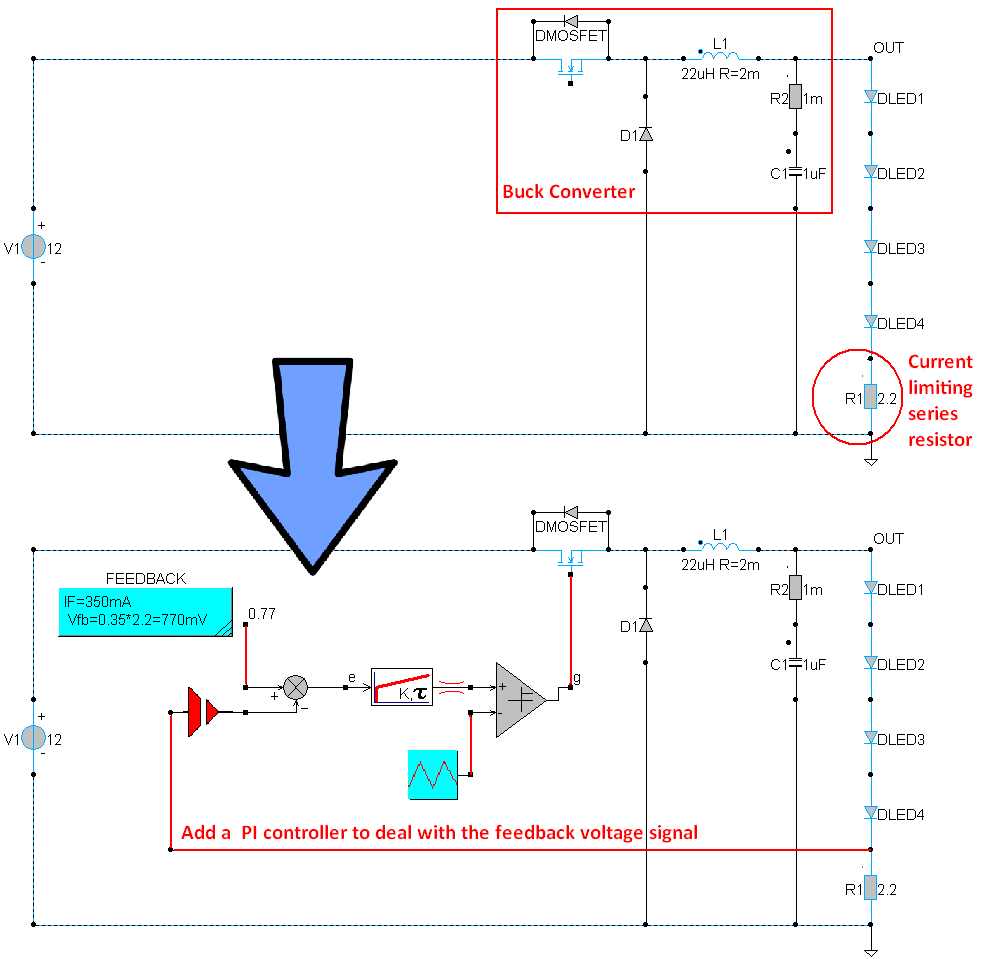To build the above schematic, the necessary components are as below. To select the components from component list, find out the location of the necessary component, left-click the component and release the mouse button, and then move your mouse cursor into the workscreen and left-click again. The parameters of each component are shown in brackets behind. Right-click components for editing the required parameters.

Using components:

• Components/Circuit/Sources/V(value=12)
• Components/Circuit/RLC/R(R1: value=2.2; R2: value=1m)
• Components/Circuit/RLC/L(value= 22uH R=2m, initial condition=0)
• Components/Circuit/RLC/C(value=1uF, initial condition=0)
• Components/Circuit/Semiconductors/D(D1: Model Parameters: Rd(on)=10m, Rd(off)=1e6, Vd(on)=0.5, BV(BreakDown)=1e6; DMOSFET: Name=DMOSFET, Model Parameters: Rd(on)=10m, Rd(off)=1e6, Vd(on)=0.7, BV(BreakDown)=1e6; DLED1: Name=DLED1, Model Parameters: Rd(on)=10m, Rd(off)=1e6, Vd(on)=0.6, BV(BreakDown)=1e6; DLED2: Name=DLED2, Model Parameters: Rd(on)=10m, Rd(off)=1e6, Vd(on)=0.6, BV(BreakDown)=1e6; DLED3: Name=DLED3, Model Parameters: Rd(on)=10m, Rd(off)=1e6, Vd(on)=0.6, BV(BreakDown)=1e6; DLED4: Name=DLED4, Model Parameters: Rd(on)=10m, Rd(off)=1e6, Vd(on)=0.6, BV(BreakDown)=1e6)
• Components/Circuit/Semiconductors/MOSFET(Model Parameters: Rds(on)=10m, Rds(off)=100k, Vds(on)=0, BVdss(Vmax)=1e6)
• Components/Blocks/NonLinear/COMP(Name=g, Parameters Block Diagram: p1=1, p2=0)

We can find the above components in the following paths:• Components/Blocks/Math/SUB(Name=e)
• Components/ Library/Control/ContinuousControllers/PILIMtau(K=100m, Max=0.95, Min=0.05, tau=5u)
• Components/ Library/Source/System/TriangleWave(Amplitude=0.501, DC_offset=0.5, Frequency=1MEG, Phase[0-360]=0, d[0..1]=0.5)
• Components/Library/Sensor/Voltage/v(Gain=1)
• Components/ Library/Electric/Ground/Ground

We can find the above components in the following paths: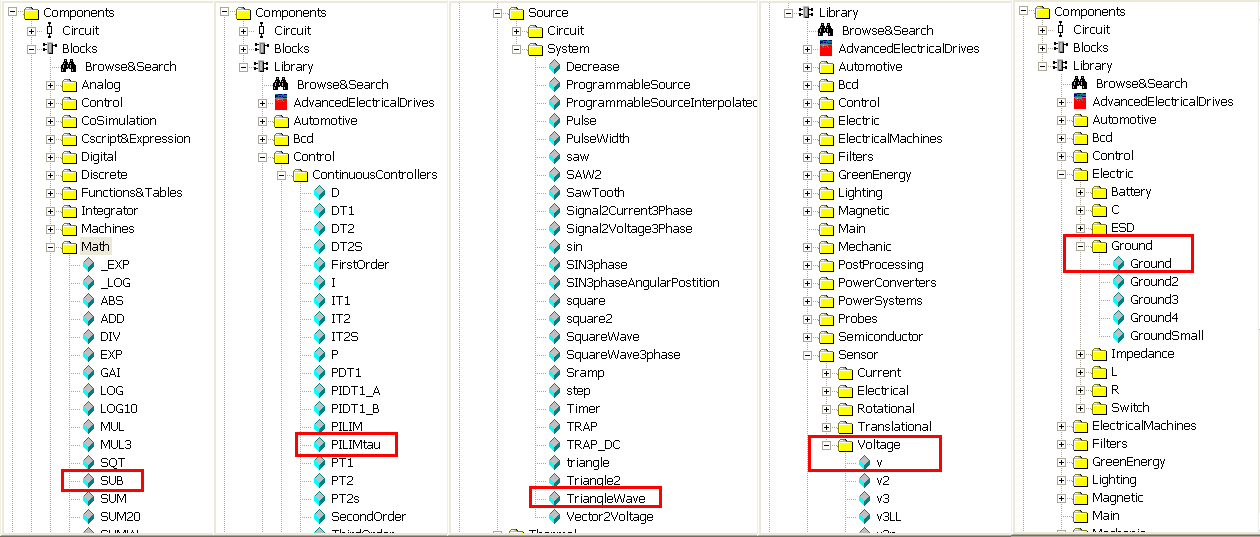• Components/Blocks/Miscellaneuous/NOTE or Insert/Note or click the short-cut buttonin the experiment bar(Text1= IF=350mA, Vfb=0.35*2.2=770mV)• Assign a label: (Click with the right mouse button on the node. Refer to Introduction tutorial 3-Simple Circuit to know how to use �label�.)
• The cathode of DLED1 � OUT which stands for the output voltage of the buck converter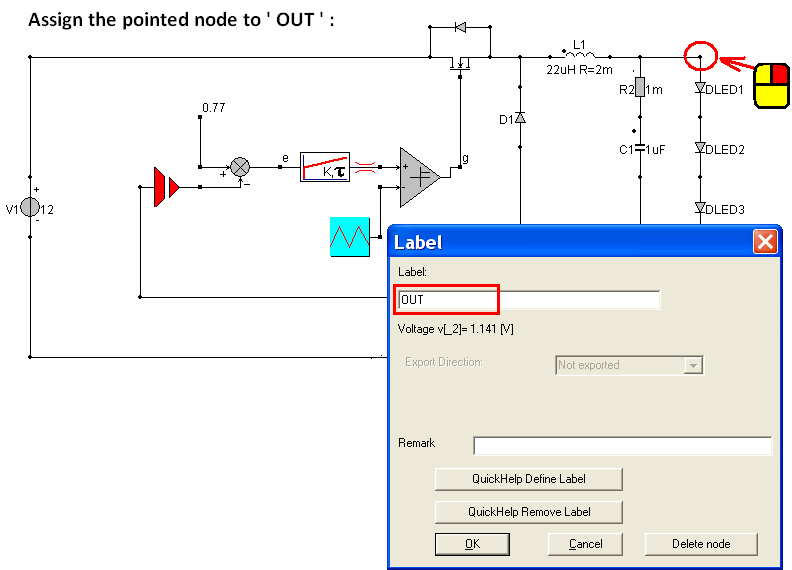• Components/Blocks/Sinks/SCOPE or Insert/Scope or click the short-cut button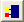in the experiment bar
• (Select two scopes and enlarge them. First scope: assign the label �OUT� to the first input trace, the current via DLED4 to the second input trace and the value �0.35� to the third input trace. Second trace: assign the output �g� of COMP to the first input trace.)
• (Notice that the output node of LED-Buck converter is a circuit node. If we want to use Scope to read the value of a circuit node, we shall change the dot of input trace to round, not the default square. To make a round dot on the input trace, we can simply use a resistor and connect its cathode or anode to the input trace. Then the input trace will become a round dot in order to get the value from the resistor. Hence we can assign a circuit node to the scope by its round dot. The default dot of Scope is square dot which is used to read the signal values from the schmeatic. You'll have a better idea from the following figure.)Simulation parameters: (Go to Simulation/Simulation Parameters or click the short-cut buttonin the menu bar)

• Screen width (Tscreen) =100u
• Integration step size (dt)=5n
• Show each 1 timestep simulation results
• Select: Wait After Screen
• Numerical Integration Method Circuit: Euler (To know the differences between the three methods, refer to Introduction Tutorial 5: Simulation Parameters)

After configuring all the necessary parameters, go to Simulation/Start simulation to execute the simulation or click the short-cut button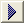in the menu bar and get the following result.The first blue curve is the output voltage of buck converter. The red curve is the current vale through the four LEDs and sky blue is the current reference value. The reference current is often set 300mA but up to 350mA.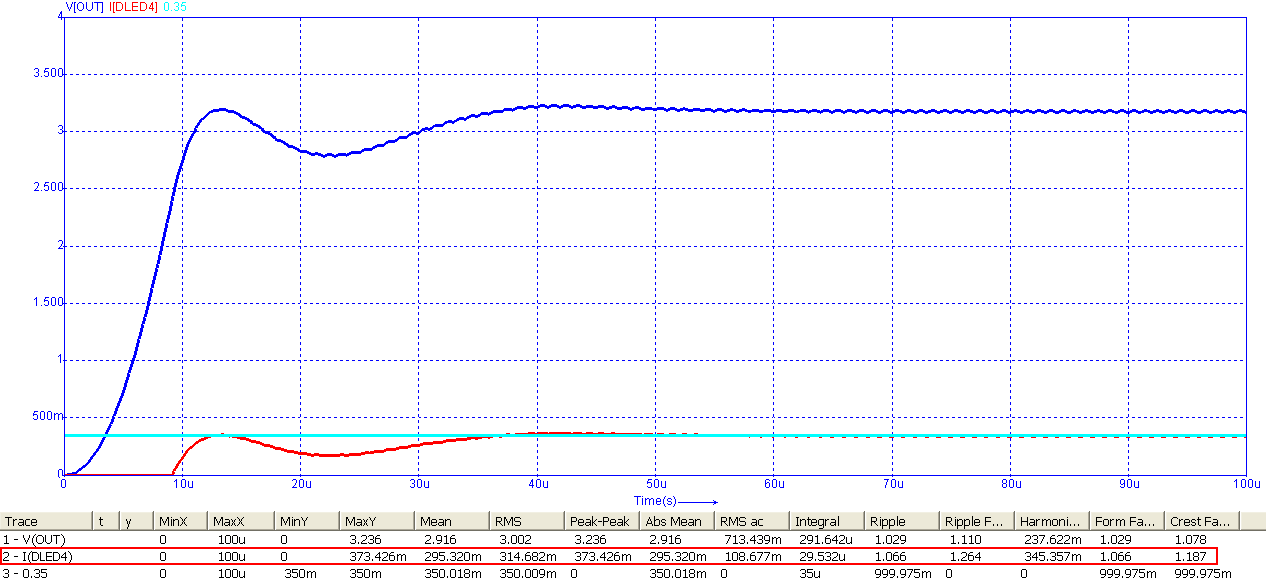The control signal from PI controller to the switch of buck converter.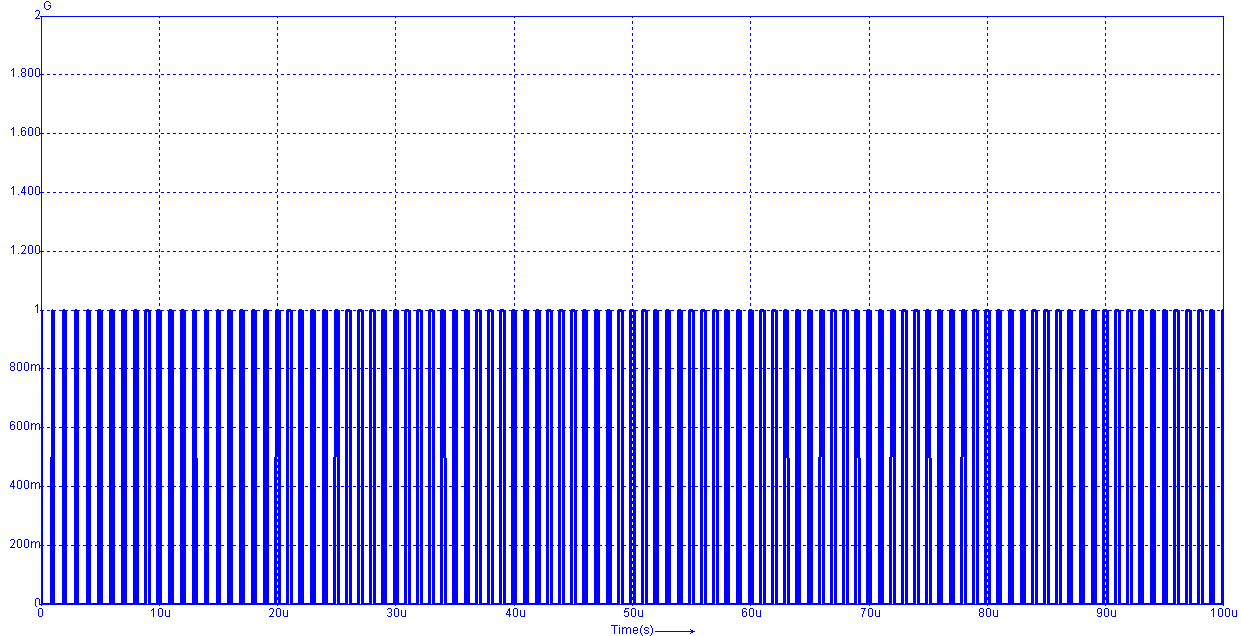For controlling the brightness of LED, we can also change the reference current to 300mA so that feedback voltage will become 0.66 V. Modify the third input trace of the first scope to 0.3 A (300mA) and re-run the simulation.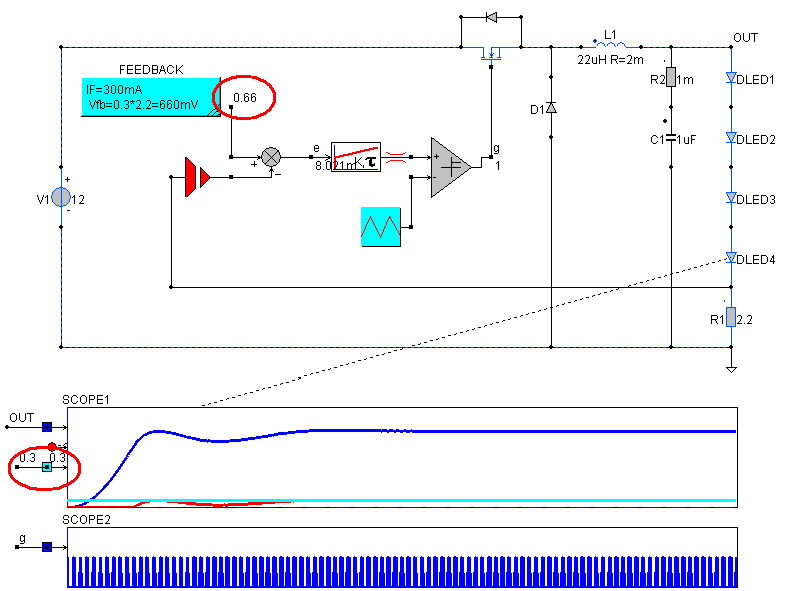Get the following result and see if the PI controller works well (restrict the current via LED according to the reference current 300mA).